# CHSE Odisha Class 11 Math Solutions Chapter 8 Permutations and Combinations Ex 8(c)

Odisha State Board Elements of Mathematics Class 11 Solutions CHSE Odisha Chapter 8 Permutations and Combinations Ex 8(c) Textbook Exercise Questions and Answers.

## CHSE Odisha Class 11 Math Solutions Chapter 8 Permutations and Combinations Exercise 8(c)

Question 1.
Compute the following :
(i) 12C3
Solution:
12C3 = $$\frac{(12) !}{3 ! 9 !}=\frac{12 \cdot 11 \cdot 10}{3 \cdot 2}$$ = 220

(ii) 15C12
Solution:
15C12 = $$\frac{(15) !}{(12) ! 3 !}=\frac{15 \cdot 14 \cdot 13}{3 \cdot 2}$$
= 5.7.13 = 455

(iii) 9C4 + 9C5
Solution:
9C4 + 9C5 = $$\frac{9 !}{4 ! 5 !}+\frac{9 !}{5 ! 4 !}$$
$$=\frac{9 \cdot 8 \cdot 7 \cdot 6}{4 \cdot 3 \cdot 2 \cdot 1}$$ × 2 = 252

(iv) 7C3 + 6C4 + 6C3
Solution:
7C3 + 6C4 + 6C3 = 7C3 + 6C4 + 6C4-1
= 7C3 + 6+1C4 = 7C3 + 7C4
(∴ ncr + nCr-1– = n+1cr)
= 7C4 + 7C4-1 = 7+1C4
= 8C4 = $$\frac{8 !}{4 !(8-4) !}=\frac{8 \cdot 7 \cdot 6 \cdot 5}{4 \cdot 3 \cdot 2 \cdot 1}$$ = 70

(v) 8C0 + 8C1 + …….. + 8c8
Solution:
8C0 + 8C1 + …….. + 8c8 = 28 = 256Question 2.
Solve :
(i) nC4 = nC11 ;
Solution:
nC4 = nC11 ;  (∴ n = 4 + 11 = 15)

(ii) 2nC3 : nC3 = 44: 5
Solution:
2nC3 : nC3 = $$\frac{44}{5}$$
⇒ $$\frac{2 n !}{2 n-3 !} / \frac{n !}{n-3 !}=\frac{44}{5}$$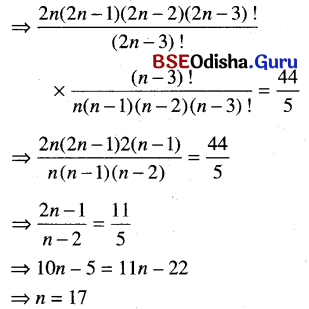Question 3.
Find n and r if nPr = 1680, nCr = 70.
Solution:
nPr = 1680, nCr = 70
∴ $$\frac{{ }^n \mathrm{P}_r}{{ }^n \mathrm{C}_r}=\frac{1680}{70}$$
or, r ! = 24 = 4!
∴ r = 4
Again, nCr = 70 or nC4 = 70
or, $$\frac{n !}{4 !(n-4) !}=70$$
or, n(n – 1) (n – 2) (n – 3)
= 70 × 4! = 7 × 10 × 4 × 3 × 2
= 8 × 7 × 6 × 5
or, n(n – 1) (n – 2) (n – 3)
or, 8(8 – 1) (8 – 2) (8 – 3)
∴ n = 8

Question 4.
How many diagonals can an n-gon(a polygon with n sides) have?
Solution:
A polygon of n – sides has n vertices.
∴ The number of st. lines joining the n-vertices is nC2.
∴ The number of diagonals is nC2 – n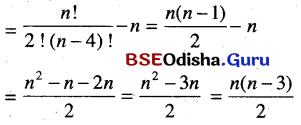Question 5.
If a set A has n elements and another set B has m elements, what is the number of relations from A to B?
Solution:
If |A| = n, |B| = m
then |A × B| = mn
∴ The number of possible subsets of
A × B = 2mn
∴ The number of relations from A to B is 2mn.Question 6.
From five consonants and four vowels, how many words consist of three consonants and two vowels?
Solution:
Words of consisting of 3 consonants and 2 vowels are to be formed from five consonants and 4 vowels.
∴ The number of ways = 5C3 × 4C2
Again, 5 letters can be arranged among themselves in 5! ways.
∴ The total number of ways
= 5C3 × 4C2 × 5! = 10 × 6 × 120 = 7200.

Question 7.
In how many ways can a committee of four gentlemen and three ladies be formed out of seven gentlemen and six ladies?
Solution:
A committee of 4 gentlemen and 3 ladies is to be formed out of 7 gentlemen and 6 ladies.
∴ The number of ways in which the committee can be formed.
7C4 × 6C3 = $$\frac{7 \cdot 6 \cdot 5}{3.2} \times \frac{6 \cdot 5 \cdot 4}{3 \cdot 2}$$ = 700

Question 8.
A bag contains 4 black and 5 white balls out of which 6 balls are drawn arbitrarily. In how many ways can this be done? Find also the number of ways such that at least 3 black balls can be drawn.
Solution:
A bag contains 4 black and 5 white balls out of which 6 balls are drawn arbitrarily.
∴ The number of ways in which balls are drawn $${ }^9 \mathrm{C}_6=\frac{9 \cdot 8 \cdot 7}{3 \cdot 2 \cdot 1}$$ = 84 as the total number of balls is 9. If at least 3 black balls are drawn, then the drawing can be made as follows.

 Black(4) White(5) 3 3 4 2

The number of ways in which at least 3 black balls are drawn
= (4C3 × 5C3) + (4C4 × 5C2)
= (4 × 10) + (1 × 10) = 50

Question 9.
How many triangles can be drawn by joining the vertices of a decagon?
Solution:
A decagon has 10 vertices and 3 noncollinear points are required to be a triangle.
∴ The number of triangles formed by the joining of the vertices of a decagon is
10C3 = $$\frac{10 !}{3 ! 7 !}=\frac{10 \cdot 9 \cdot 8}{3 \cdot 2 \cdot 1}$$ = 120

Question 9.
How many triangles can be drawn by joining the vertices and the center of a regular hexagon?
Solution:
A regular hexagon has six vertices. Triangles are to be formed by joining the vertices and center of the hexagon. So there is a total of 7 points. So the number of triangles formed.
7C3 = $$\frac{7 !}{3 ! 4 !}=\frac{7 \cdot 6 \cdot 5}{3 \cdot 2 \cdot 1}$$ = 35
As the hexagon has 3 main diagonals, which pass through the center hence can not form 3 triangles.
∴ The required number of triangles 35 – 3 = 32

Question 11.
Sixty points lie on a plane, out of which no three points are collinear. How many straight lines can be formed by joining pairs of points?
Solution:
Sixty points lie on a plane, out of which no. 3 points are collinear. A straight line required two points. The number of straight lines formed by joining 60 points is
60C2 = $$\frac{60 !}{2 \times 58 !}=\frac{60 \times 59}{2}$$ = 1770

Question 12.
In how many ways can 10 boys and 10 girls sit in a row so that no two boys sit together?
Solution:
10 boys and 10 girls sit in a row so that no two boys sit together. So a boy is to be seated between two girls or at the two ends of the row. So the boys are to be sitted in 11 positions in 11C10 ways. Again 10 boys and 10 girls can be arranged among themselves in 10! and 10! ways respectively.
∴ The total number of ways = 11C10 × 10! × 10! = (11)! × (10)!

Question 13.
In how many ways can six men and seven girls sit in a row so that the girls always sit together?
Solution:
Six men and seven girls sit in a row so that the girls always sit together. Considering the 7 girls as one person, there are a total of 7 persons who can sit in 7! ways. Again the 7 girls can be arranged among themselves in 7! ways.
∴ The total number of arrangements
= 7! × 7!
= (7!)2Question 14.
How many factors does 1155 have that are divisible by 3?
Solution: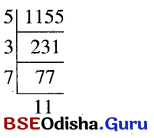∴ In order to be a factor of 1155 divisible by 3, we have to choose one or two of 5, 7, and 11 along with 3 or 3 alone.
∴ The number of ways = 3C1 + 3C2 + 3C0 = 23 – 1 = 7
∴ The number of factors is 7 excluding 1155 itself.

Question 15.
How many factors does 210 have?
Solution: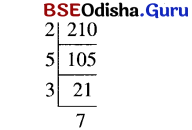∴ We can choose at least one 2, 3, 5, or 7  to be a factor of 210.
∴ The number of factors.
= 4C1 + 4C2 + 4C3 + 4C4 = 24 – 1 = 15
∴ The number of factors is 210 is 15. (Including 215 itself and excluding 1).

Question 16.
If n is a product of k distinct primes what is the total number of factors of n?
Solution:
n is a product of k distinct primes.
∴ In order to be a factor, of n, we have chosen at least one of k distinct primes.
∴ The number of ways = kC1 + kC2 + ……… kCk-1 = 2k  – 1 – 1
∴ The number of factors of n is 2k – 2.
(Excluding 1 as 1 is not prime. It is also not include n.)

Question 17.
If m has the prime factor decomposition P1r1, P2r2 ….. Pnrn, what is the total number of factors of m (excluding 1)?
Solution:
m has the prime factor decomposition P1r1, P2r2 ….. Pnrn,
∴ m = P1r1, P2r2 ….. Pnrn,
P1 is a factor of m which occurs r1 times. Each of the factors P1r1 will give rise to (r1 + 1) factors.
Similarly
P2r2 gives (r2 + 1) factors and so on.
∴ The total number of factors (r1 + 1) (r2 + 1) ….. (rn + 1) – 1 (including m).

Question 18.
If 20! were multiplied out, how many consecutive zeros would it have on the right?
Solution:
If 20! were multiplied out, then the number of consecutive zeros on the right is 4. due to the presence of 4 x 5, 10, 14 x 15,20.

Question 19.
How many factors of 10,000 end with a 5 on the right?
We have 1000 = 24 × 54 The factors of 10000 ending with 5 are 5, 5 × 5 = 25, 5 × 5 × 5 = 125
5 × 5 × 5 × 5 = 625
∴ There are 4 factors ending With 5.

Question 20.
A man has 6 friends. In how many ways can he invite two or more to a dinner party?
Solution:
A man has 6 friends. He can invite 2 or more of his friends to a dinner party.
∴ He can invite 2, 3, 4, 5, or 6 of his friends in
6C2 + 6C3 + 6C4 + 6C5 + 6C6 = 266C06C1
= 64 – 7 = 57 ways.

Question 21.
In how many ways can a student choose 5 courses out of 9 if 2 courses are compulsory?
Solution:
A student is to choose 5 courses out of 9 in which 2 courses are compulsory. as 2 courses are compulsory, he is to choose 3 courses out of 7 courses in 7C3 = 35 ways.

Question 22.
In how many ways can a student choose five courses out of the courses? C1, C2, …………. C9 if C1, C2 are compulsory and C6, C8 cannot be taken together?
Solution:
A student chooses five courses out of the courses? C1, C2, …………. C9 if C1, C2 are compulsory and C6, C8 cannot be taken together.
∴ He is to choose 3 courses out of C3, C4, ………… ,C8, C9.
Without taking any restrictions 3 courses out of C3, C4, …… C9, i.e. from 7 courses in 7C3 ways. If C6, C8 are taken together then one course only to be choosen from C3, C4, C5, C7, C9 by 5C1 ways. Hence required number of ways.
= 7C35C1
= $$\frac{7 \times 6 \times 5}{3 \times 2 \times 1}$$ – 5
= 35 – 5 = 30 ways.Question 23.
A cricket team consisting of 11 players is to be chosen from 8 batsmen and 5 bowlers. In how many ways can the team be chosen so as to include at least 3 bowlers?
Solution:
A cricket team consisting of 1 1 player is to be chosen from 8 batsmen and 5 bowlers, as to include at least 3 bowlers.
The selection can be made as follows :

 Batsmen(8) Bowlers(5) 8 3 7 4 6 5

The number of selections is
(8C8 × 5C3) + (8C7 × 5C4) + (8C6 × 5C5)
= (1 × 10) + (8 × 5) + (28 × 1)
= 10 + 40 + 28 = 78

Question 24.
There are n + r points on a plane out of which n points lie on a straight line L and out of the remaining r points that lie outside L, no three points are collinear. What is the number of straight lines that can be formed by joining pairs of their points?
Solution:
There are n + r points on a plane out of which n points are collinear and out of which r points are not collinear.
∴ We can form a straight line by joining any two points.
n-collinear points form one line and r-non-collinear points form rC2 lines.
Again, each of the r non-collinear points when joined to each of the noncollinear points, forms n lines.
∴ The number of such limes is r x n.
∴ The total number of lines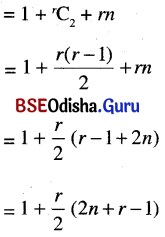Question 25.
There are 10 books in a shelf with different titles; five of these have red covers and others have green covers. In how many ways can these be arranged so that the red books are placed together?
Solution:
There are 10 books in a shelf with different titles, 5 of these are red covers and others are green covers considering 5 red-covered books as one book, we have a total of 6 books which can be arranged in 6! ways. The five red cover books are arranged among themselves in 5! ways.
∴ The total number of arrangements
= 5! x 6!